Journal of World Economic Research
Volume 4, Issue 5, October 2015, Pages: 109-114

Descriptive Study of 2009-2013 China Area per Capita GDP

Renhao Jin, Fang Yan, Jie Zhu

School of Information, Beijing Wuzi University, Beijing, China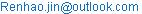(Renhao Jin)

Renhao Jin, Fang Yan, Jie Zhu. Descriptive Study of 2009-2013 China Area per Capita GDP.Journal of World Economic Research.Vol.4, No. 5, 2015, pp. 109-114. doi: 10.11648/j.jwer.20150405.11

Abstract: This paper studied area level per capita GDP data from 2009 to 2013 in China. The bar chart, bubble chart and map chart are used to display a growth trend on area per capita GDP. It is pointed out that areas with higher Per Capita GDP have relative lower growth rate on Per Capita GDP. Moran's I coefficients and Geary's C coefficients are used to measure the Spatial autocorrelation in the Per capita GDP data. The results of Moran's I coefficient and Geary's c coefficients test showed that global spatial autocorrelation are accepted, while local spatial autocorrelation are rejected.

Keywords: China GDP, Area per Capita GDP, Spatial Analysis

Contents

1. Introduction

Gross domestic product (GDP) is defined by the Organization for Economic Co-operation and Development (OECD) as "an aggregate measure of production equal to the sum of the gross values added of all resident, institutional units engaged in production (plus any taxes, and minus any subsidies, on products not included in the value of their outputs)." The more familiar use of GDP estimates is to calculate the growth of the economy from year to year (and recently from quarter to quarter). The pattern of GDP growth is held to indicate the success or failure of economic policy and to determine whether an economy is 'in recession'.

Recently years, China’s total GDP has ranked in the second in the world, and some economists predict that China’s GDP will catch up United States’ in 15 years. China economic has attracted many researchers in the world. However, China Per Capita GDP are still relative lower than that of western countries. This paper does a description study on China Per Capita GDP. The data used in this paper are obtained from China Statistical Yearbook, published by National Bureau of Statistics of China.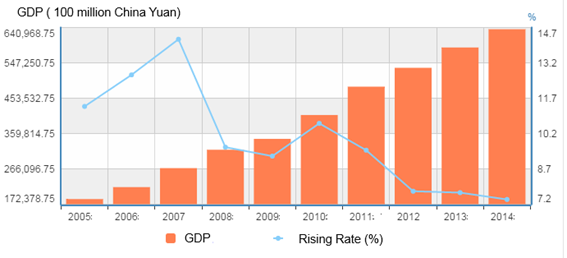Figure 1. The China GDP and its increasing rate from 2005 to 2014.

There are 28 provinces and 4 municipalities directly under the Central Government of China mainland. Beijing, Shanghai, Tianjin, Chongqing are the four municipalities, and Beijing and Shanghai are two very important cities in China and the World.Beijing is located in the north of China, which is the center of North China and capital of China. Shanghai is in the east of China, and the areas around Shanghai is the richest part in China.

As shown in Figure 1, from 2005 to 2014, China GDP is always in rising status, although it increasing rate is decreasing. However, in 2014, its increasing rate is still about 7.2%, which is relative high figure comparing 2.4% of United Status and negative increasing rate in some Europe countries. In 2005, China GDP is only about 17237.8 Billion (China Yuan), but in 2014, it is almost 4 times more. With this high speed development, in 2007 and 2010, China catch up with Germany and Japan respectively. At the same time China’s population keeps relative stable, so the rising rate of Per Capita GDP is similar to that of total GDP.

2. The Area per Capita GDP

The area level per capita data are listed in the table 1, and it contains 5 years data from 2009 to 2013. Similar to China GDP overall increasing trend, Per Capita GDP in all areas is increasing. As shown in Figure 2, there are 8, 4, and 1 areas in the first chart (marked as 12000) in year 2009, 2010, 2011 respectively, but in year 2012 and 2013 no area is in that chart. At the same time, the charts with larger marked values all have experienced increasing process but with different extent. Just as reported by articles in Medias, Chinese citizens are becoming richer and richer. With this phenomena, Chinese people spend more and more money for shopping in the world.

Table 1. The Per Capita GDP (China Yuan) for 31 areas in China mainland from 2009 to 2013.

 No. Areas Per Capita GDP (China Yuan) 2009 2010 2011 2012 2013 1 Beijing 66940 73856 81658 87475 93213 2 Tianjin 62574 72994 85213 93173 99607 3 Hebei 24581 28668 33969 36584 38716 4 Shanxi 21522 26283 31357 33628 34813 5 Neimenggu 39735 47347 57974 63886 67498 6 Liaoning 35149 42355 50760 56649 61686 7 Jilin 26595 31599 38460 43415 47191 8 Heilongjiang 22447 27076 32819 35711 37509 9 Shanghai 69164 76074 82560 85373 90092 10 Jiangsu 44253 52840 62290 68347 74607 11 Zhejiang 43842 51711 59249 63374 68462 12 Anhui 16408 20888 25659 28792 31684 13 Fujian 33437 40025 47377 52763 57856 14 Jiangxi 17335 21253 26150 28800 31771 15 Shandong 35894 41106 47335 51768 56323 16 Henan 20597 24446 28661 31499 34174 17 Hubei 22677 27906 34197 38572 42613 18 Hunan 20428 24719 29880 33480 36763 19 Guangdong 39436 44736 50807 54095 58540 20 Guangxi 16045 20219 25326 27952 30588 21 Hainan 19254 23831 28898 32377 35317 22 Chongqing 22920 27596 34500 38914 42795 23 Sichuan 17339 21182 26133 29608 32454 24 Guizhou 10971 13119 16413 19710 22922 25 Yunnan 13539 15752 19265 22195 25083 26 Xizang 15295 17319 20077 22936 26068 27 Shanxi 21947 27133 33464 38564 42692 28 Gansu 13269 16113 19595 21978 24296 29 Qinghai 19454 24115 29522 33181 36510 30 Ningxia 21777 26860 33043 36394 39420 31 Xinjiang 19942 25034 30087 33796 37181Figure 2. The bar chart of area Per Capita GDP. The horizontal axis is the frequency and the longitudinal axis are the GDP values (Unit: China Yuan). The bar charts are divided into 5 groups by the years.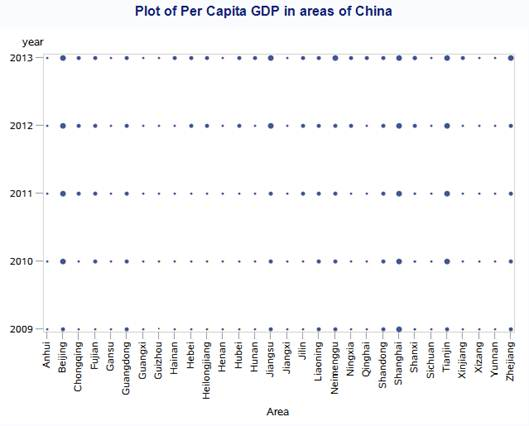Figure 3. The Bubble Chart of area Per Capita GDP. The horizontal axis is all the area list, and the longitudinal axis are the years from 2009 to 2013. The size of bubble is proportional to the value scale of Per Capita GDP (Unit: China Yuan) at the corresponding area and year.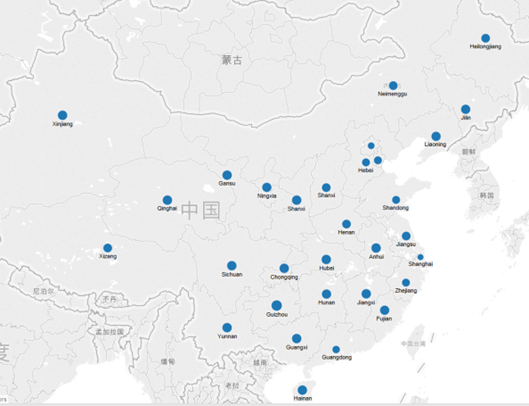Figure 4. The map of average growth rate on Per Capita GDP. In this map, all areas are marked by bubbles. The size of bubble is proportional to the value scale of average growth rate of Per Capita GDP at the corresponding area.

The Bubble Chart of area Per Capita GDP is shown in Figure 3.The size of bubble is proportional to the value scale of Per Capita GDP (Unit: China Yuan) at the corresponding area and year. It can be seen from the plot that the rank of Per Capita GDP of each area keeps stable, although different areas have different growth rate. For the areas like Beijing and Shanghai, the bubbles from 2009 to 2013 have little changes in the size, which means its growth rate is at a small scale. However, for the areas like Jiangsu and Heilongjiang, bubbles have a significant changes in size, and it is because these areas have a high growth rate.

The average Per Capita GDP map is shown in Figure 5. The average Per Capita GDPare calculated by the mean value of Per Capita GDP value from 2009 to 2012. In this figure, all areas are marked by bubbles. The size of bubble is proportional to the value scale of average value of Per Capita GDP at the corresponding area. It can be seen that the areas in the east China along the coast line and Beijing have relative large bubbles. This phenomena is opposite to that is shown in Figure 4 as these areas have relative small bubbles. However, it is very reasonable that the higher Per Capita GDP the more difficult to increase it.

3. The Spatial Autocorrelation of Area per Capita GDP

In this section, the spatial autocorrelation among the Per Capita GDP data is investigated. The average Per Capita GDP for all areas is the target objective variable. It can be seen from the Figure 5 that large bubbles are distributed along the coastline in the east of China. The Moran’s I coefficient and Geary's Ccoefficient are used to measure the spatial autocorrelation.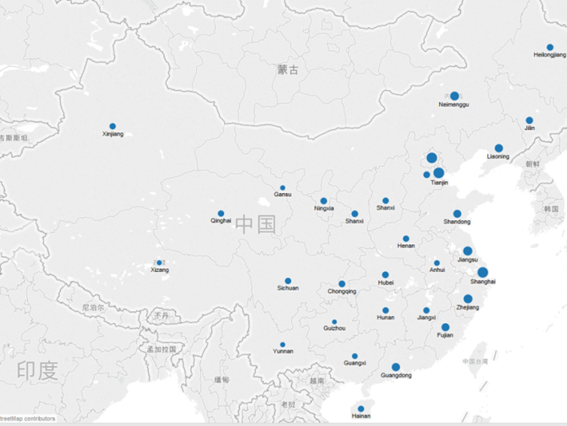Figure 5. The map of average value of Per Capita GDP. In this map, all areas are marked by bubbles. The size of bubble is proportional to the value scale of average value of Per Capita GDP at the corresponding area.

The Moran’s I coefficient are defined as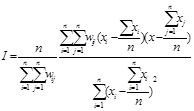,

and the Geary's c coefficient are calculated as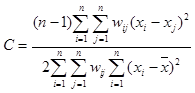.

In statistics, Moran's I is a measure of spatial autocorrelation developed by Patrick A.P. Moran. Like autocorrelation, spatial autocorrelation means that adjacent observations of the same phenomenon are correlated. Negative (positive) values indicate negative (positive) spatial autocorrelation. Moran's IValues range from − 1 (indicating perfect dispersion) to + 1 (perfect correlation). A zero values indicates a random spatial pattern. For statistical hypothesis testing, Moran's I values can be transformed to Z-scores in which values greater than 1.96 or smaller than − 1.96 indicate spatial autocorrelation that is significant at the 5% level. The Z-scores transformation can be easily written as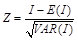.

Geary's C is also a measure of spatial autocorrelation or an attempt to determine if adjacent observations of the same phenomenon are correlated. Geary's C is inversely related to Moran's I, but it is not identical. Moran's I is a measure of global spatial autocorrelation, while Geary's C is more sensitive to local spatial autocorrelation.Geary's C is also known as Geary's contiguity ratio or simply Geary's ratio.The value of Geary's C lies between 0 and 2. 1 means no spatial autocorrelation. Values lower than 1 demonstrate increasing positive spatial autocorrelation, whilst values higher than 1 illustrate increasing negative spatial autocorrelation.

The Moran's I coefficient and Geary's c coefficient are calculated and shown in table 2. It can be seen that average area Per Capita GDP in China are displayed positive autocorrelation both from Moran's I coefficient and Geary's c coefficient, which means the per capita GDP data in the nearby areas are tended to be similar. However, at the same time it is also noticed that only weak positive autocorrelation are found in the data, as the Moran's I coefficient are close to 0, and Geary's c coefficient are close to 1.The p-value of the spatial autocorrelation test are 0.0078 and 0.1311 for Moran's I coefficient and Geary's c coefficients respectively, thus spatial autocorrelations of area Per Capita GDP value are accepted by Moran's I coefficient but rejected by Geary's c coefficients test. Recalling that Moran's I is a measure of global spatial autocorrelation, while Geary's C is more sensitive to local spatial autocorrelation, it can be seen from Figure 5 that spatial autocorrelation can be found overall but cannot be found in many local areas, for example, the parts away from coastline.

Table 2. The Moran's I coefficient and Geary's c coefficient computed onarea Per Capita GDP data in China.

 Spatial autocorrelation coefficients Assumption Coefficient Observed Pr > |Z| Randomization Moran's I 0.196 0.0078 Randomization Geary's C 0.773 0.1311

4. Conclusion

This paper firstly make a descriptive study on area level per capita GDP data in China. A growth trend on per capita GDP can be found in all areas in China. However, the disequilibrium is also noticed in different areas. It is also pointed out that areas with higher Per Capita GDP have relative lower growth rate on Per Capita GDP. Then, Moran's I coefficients and Geary's C coefficients are used to measure the Spatial autocorrelation in the Per capita GDP data. Weak positive autocorrelation are found both from Moran's I and Geary's c coefficients. Moreover, from the results of Moran's I coefficient and Geary's c coefficients test, global spatial autocorrelation are accepted, while local spatial autocorrelation are rejected.

Acknowledgements

This paper is funded by the project of National NaturalScience Fund, Logistics distribution of artificial orderpicking random process model analysis and research (Projectnumber: 71371033); and funded by intelligent logisticssystem Beijing Key Laboratory (No.BZ0211); and funded by scientific-research bases---Science & TechnologyInnovation Platform---Modern logistics information andcontrol technology research (Project number:PXM2015_014214_000001); University Cultivation FundProject of 2014-Research on Congestion Model andalgorithm of picking system in distribution center(0541502703).

References

1. Anselin, L. (1995). Local indicators of spatial association – LISA. Geographical Analysis 27, 93--115.
2. Chegut, A.M., Eichholtz, P.M.A, Rodrigues, P. J. M. (2015). Spatial Dependence in International Office Markets. The Journal of Real Estate Finance and Economics. Volume 51, Issue 2, pp 317-350.
3. Besag, J. (1974). Spatial interaction and the statistical analysis of lattice systems. Journal of the Royal Statistical Society B 36, 192--225.
4. Dawson, Graham (2006). Economics and Economic Chenge. FT / Prentice Hall. p. 205. ISBN 9780273693512.
5. Getis, A. and Ord, J. K. (1992). The analysis of spatial association by use of distance statistics. Geographical Analysis 24, 189--206.
6. Griffith, D. (1992). What is spatial autocorrelation? Reflections on the past 25 years of spatial statistics. l’Espace Ge´ographique 21,265--280.
7. Griffith, D. (1996). Spatial autocorrelation and eigenfunctions of thegeographic weights matrix accompanying geo-referenced data. The Canadian Geographer 40, 351--367.
8. Griffith, D. and Chun, Y. (2015). Spatial Autocorrelation in Spatial Interactions Models: Geographic Scale and Resolution Implications for Network Resilience and Vulnerability. Networks and Spatial Economics.
9. "GDP (Official Exchange Rate)". CIA World Factbook. Retrieved June 2, 2012.
10. Liu, Y., Schen, C., Li, Y. (2015). Differentiation regularity of urban-rural equalized development at prefecture-level city in China. Journal of Geographical Sciences.
11. Mardia, K. and Marshall, R. (1984). Maximum likelihood estimation ofmodels for residual covariance in spatial regression. Biometrika 71,135--146.
12. Mohebbi, M., Wolfe, R., Jolley, D. (2011). A poisson regression approach for modelling spatial autocorrelation between geographically referenced observations. BMC Medical Research Methodology.
13. Peck, G.(2013). Tableau 8: The Official Guide by Peck, George (2013) Paperback. McGraw-Hill Osborne Media.
14. Richardson, S. and He´ mon, D. (1981). On the variance of the samplecorrelation between two independent lattice processes. Journal ofApplied Probability 18, 943--948.
15. Tiefelsdorf, M. and Boots, B. (1995). The exact distribution of Moran’s I.Environment and Planning.A 27, 985--999.
16. SAS Institute Inc, (2008). SAS/STAT® 9.2 User’s Guide: The variogram Procedure (Book Excerpt). NC: SAS Institute Inc, Cary.

 Contents 1. 2. 3. 4.
Article ToolsAbstractPDF(1346K)